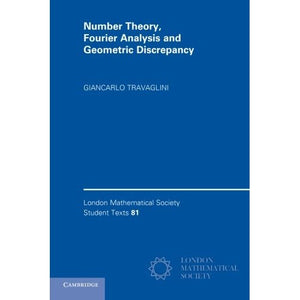># Number Theory, Fourier Analysis and Geometric Discrepancy (London Mathematical Society Student Texts)

• £12.29
• Save £10

Giancarlo Travaglini
Cambridge University Press, 6/12/2014
EAN 9781107619852, ISBN10: 1107619858

Paperback, 252 pages, 22.9 x 15.2 x 1.4 cm
Language: English

The study of geometric discrepancy, which provides a framework for quantifying the quality of a distribution of a finite set of points, has experienced significant growth in recent decades. This book provides a self-contained course in number theory, Fourier analysis and geometric discrepancy theory, and the relations between them, at the advanced undergraduate or beginning graduate level. It starts as a traditional course in elementary number theory, and introduces the reader to subsequent material on uniform distribution of infinite sequences, and discrepancy of finite sequences. Both modern and classical aspects of the theory are discussed, such as Weyl's criterion, Benford's law, the KoksmaÃ¢â‚¬â€œHlawka inequality, lattice point problems, and irregularities of distribution for convex bodies. Fourier analysis also features prominently, for which the theory is developed in parallel, including topics such as convergence of Fourier series, one-sided trigonometric approximation, the Poisson summation formula, exponential sums, decay of Fourier transforms, and Bessel functions.

Part I. Elementary Number Theory
1. Prelude
2. Arithmetic functions and integer points
3. Congruences
4. Quadratic reciprocity and Fourier series
5. Sums of squares
Part II. Fourier Analysis and Geometric Discrepancy
6. Uniform distribution and completeness of the trigonometric system
7. Discrepancy and trigonometric approximation
8. Integer points and Poisson summation formula
9. Integer points and exponential sums
10. Geometric discrepancy and decay of Fourier transforms
11. Discrepancy in high dimension and Bessel functions
References
Index.